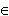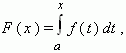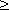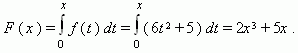# Integral with variable upper limit of integration

Let f ( x ) be a continuous function, given in a segment [ a , b ], then for any x[ a , b ] the functionexists. This function is given as an integral with variable upper limit of integration in the right-hand part of the equality.

All rules and properties of a definite integral apply to an integral with variable upper limit of integration.

 E x a m p l e . A variable force acting on a linear way changes in the law: f ( x ) = 6 x 2 + 5 at x0 . What law does a work of this force change in ?

 S o l u t i o n. A work of the force f ( x ) on a segment [ 0 , x ] of linear way is equal to:Thus, the work changes in the law: F ( x ) = 2 x 3 + 5 x .

According to the definition of an integral with variable upper limit of integration or the function F ( x ) and known properties of an integral it follows that at x[ a , b ]

F' ( x ) = f ( x ) .

Check this property using the above mentioned example.